# Accounting for partnership firms

Accounting for partnership firms fundamentals , comprises of understanding, various issues and situations that may arise in connection with partnership business, how the calculations are to be madein respect of such situations from and accounting perspective, what would be the journal entries that would be made in the books of accounts of partnership and other matters incidental thereto. These are discussed under various headings as under : –

## Commission to Partners before charging such commission & after charging commission

### Accounting for partnership firms fundamentals – Question 1: –

A and B are partners in a firm. A is to get a commission of 10 % of net profit before charging any commission. B is to get a commission of 10 % of net profit after charging all commission. Net profit before charging any commission was Rs 110000 . Calculate the Commission of A and B  ?

Explanation : –

A s commission as percentage of net profit before charging such commission
= Net Profit before Commission X Rate of Commission
100
= 110000 X 10 = 11000
100
B s commission as percentage of net profit after charging such commission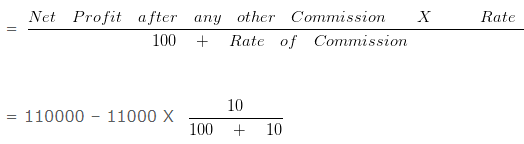= 99000 X 10/100

= 9000

## Commission to partner based on % of net profit

### Accounting for partnership firms fundamentals – Question 2 : –

A and B are partners in a firm. A is to get a commission of 12 % of net profit before charging any commission. Net profit before charging any commission was Rs. 60000 .Commission of A will be :

Explanation : –

A s commission as percentage of net profit before charging such commission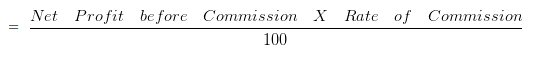= 60000 X 12/100 = 7200

## Distributable profits when loan given by partner

### Accounting for partnership firms fundamentals – Question 3 : –

A and B are partners sharing profits and losses in the ratio of 3 : 2 with capitals of Rs. 70000 and 50000 respectively.On 1st October 2015 A and B granted loans of Rs. 100000 and 50000 6300 .The total amount of distributable profits will be:

Explanation : –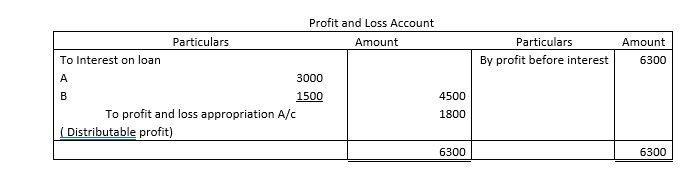Total month in a year = 12
Total months to which loan was outstanding during the year = 6

Interest on A s loan = 100000 X 6/100 X 6/12 = 3000

Interest on B s loan = 50000 X 6/100 X 6/12 = 1500

## Entry for transferring Profit to General Reserve

### Accounting for partnership firms fundamentals – Question 4 : –

The firm ABC earned a profit of Rs. 300000 during the year ending on 31st March 2015 . 11 % of this profit was to be transferred to general reserve. The necessary journal entry for the same will be:

Explanation : –

Amount to be transferred to general reserve = 300000 X 11/100 = 33000

Profit and loss appropriation A/c Dr 33000
To General Reserve A/c 33000

## Interest on additional capital introduced by partner

### Accounting for partnership firms fundamentals – Question 5 : –

A and B are partners having capital balances as Rs. 50000 and 50000 on 01-Jan-2015 . A introduced further capital of Rs. 10000 on 01-Aug-2015 and another 30000 on 15-Nov-2015 . Interest on capital to be allowed to A assuming the rate of interest to be 6 % per annum will be:

Explanation : –

Calculation of interest on capital to be allowed to A
On Rs. 50000 for full year = 50000 X 6% = 3000

On Rs. 10000 for 5 months = 10000 X 6% X 5/12 = 250

On Rs. 30000 for 1.5 months = 30000 X 6% X 1.5/12 = 225

=3475

### Accounting for partnership firms fundamentals – Question 6 : –

A and B started a partnership firm on 1st April 2017 with capitals of Rs. 200000 and Rs. 250000 respectively. On 1st January 2018 ,they decided that their capitals should be Rs . 100000 each. The necessary adjustments in the capitals are made by introducing or withdrawing cash.Interest on capital is to be allowed @ 10 % per annum. Interest on partners capital will be :

Explanation : –

Calculation of interest on capital
For A

Interst on capital from 1st April 2017 to 1st January 2018 = 200000 X 10% X 9/12

= 15000

Interst on capital from 1st January 2017 = 100000 X 10% X 3/12

= 2500
Total interst for the year = 15000 + 2500
= 17500
For B

Interst on capital from 1st April 2017 to 1st January 2018 = 250000 X 10% X 9/12

= 18750

Interst on capital from 1st January 2017 = 100000 X 10% X 3/12

= 2500

Total interst for the year = 18750 + 2500
= 21250 Rs.

## Interest on capital when closing capital given

### Accounting for partnership firms fundamentals – Question 7 : –

C and D are partners sharing profits and losses in the ratio of 2 : 1 .Their capitals at the end of the financial year 2016 – 2017 were Rs. 200000 and Rs. 150000 .During the year 2016 – 2017 , C s drawings were Rs. 10000 .Profit before charging interest on capital for the year was Rs. 18000 which was duly credited to their accounts. .Interest on capital of C @ 10 % per annum for the year 2016 – 2017 will be:

Explanation : –

Calculation of capital at the beginning of the year
Capital at the end = 200000 Rs.
Add: Drawings = 10000 Rs.
= 210000 Rs.
Less: profit credited for the year = 12000 Rs.
= 198000 Rs.
Less: Additional capital introduced =0
Capital in the beginning = 198000 Rs.
Interest on C s capital = 198000 X 10% = 19800

## Interest on Drawing- withdrawn at month’s end

### Accounting for partnership firms fundamentals – Question 8 : –

What will be the interest on drawings of A @ 10 % per annum if A withdrew Rs. 10000 per month in the end of every month.

Explanation : –

Per month drawing of A = 10000
Total months in a year = 12
Total drawings made by A during the year = 10000 X 12
= 120000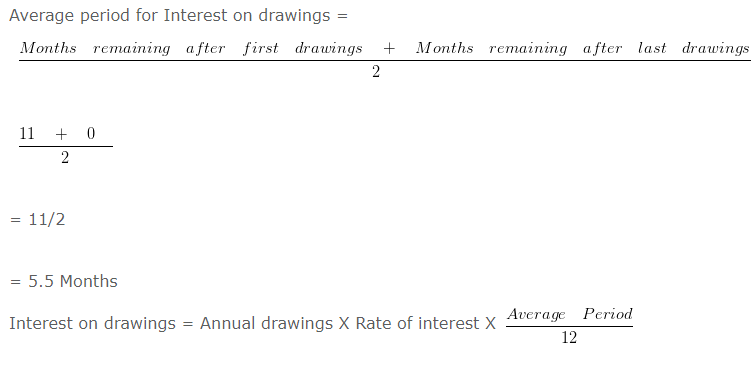= 120000 X 10% X 5.5/12

= 5500

## Interest on drawings-unequal amount-irregular intervals

### Accounting for partnership firms fundamentals – Question 9 : –

A is a partner in a firm. His drawings during the year given below for the year 2016 . Interests on drawings to be charged @ 10 % per annum.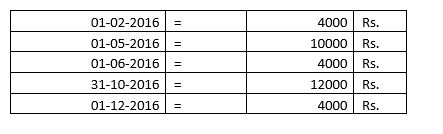The interest on A’s drawings for the year will be:

Explanation : –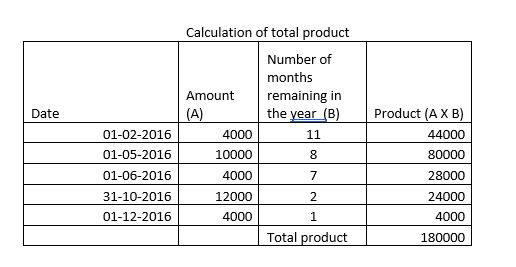Interest on Drawings = Total of Product X Rate of Drawings X 1/12

= 180000 X 10% X 1/12

= 1500 Rs.

## Interest on Drawings-withdrawn at 6 month’s end

### Accounting for partnership firms fundamentals – Question 10 : –

What will be the interest on drawings of A @ 15 % per annum if A withdrew Rs. 10000 per month in the end of every month for 6 months.

Explanation : –

Per month drawing of A = 10000
Total months in a period = 6
Total months in a year = 12
Total drawings made by A during the period = 10000 X 6
= 60000

Average period for Interest on drawings =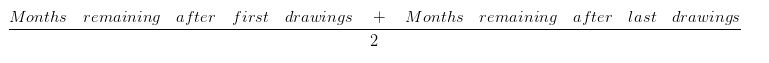= (5+0)/2

= 5/2

= 2.5 Months

Interest on drawings = Annual drawings X Rate of interest X (Average Period)/12

= 60000 X 15% X 2.5/12

= 1875

## Interest on Drawings-withdrawn at 6month’s beginning

### Accounting for partnership firms fundamentals – Question 11 : –

What will be the interest on drawings of A @ 12 % per annum if A withdrew Rs 10000 per month in the beginning of every month for 5 months.

Explanation : –

Per month drawing of A = 10000
Total months in a period = 5
Total months in a year = 12
Total drawings made by A during the period = 10000 X 5
= 50000

Average period for Interest on drawings == (6+1)/2

= 7/2

= 3.5 Months

Interest on drawings = Annual drawings X Rate of interest X (Average Period)/12

= 50000 X 12% X 3.5/12

= 1750

## Interest on Drawings-withdrawn at 6month’s mid

### Accounting for partnership firms fundamentals – Question 12 : –

What will be the interest on drawings of A @ 10 % per annum if A withdrew Rs. 10000 per month in the middle of every month for 6 months.

Explanation : –

Per month drawing of A = 10000
Total months in a period = 6
Total months in a year = 12
Total drawings made by A during the period = 10000 X 6
= 60000

Average period for Interest on drawings == (5.5+0.5)/2

= 6/12

= 3 Months

Interest on drawings = Annual drawings X Rate of interest X (Average Period)/12

= 60000 X 10% X 3/12

= 1500

## Interest on Drawings-withdrawn at 9 month’s beginning

### Accounting for partnership firms fundamentals – Question 13 : –

What will be the interest on drawings of A @ 10 % per annum if A withdrew Rs. 7000 per month in the beginning of every month for 9 months.

Explanation : –

Per month drawing of A = 7000
Total months in a period = 9
Total months in a year = 12
Total drawings made by A during the period = 7000 X 9
= 63000

Average period for Interest on drawings == (9+1)/2

= 10/2

= 5 Months

Interest on drawings = Annual Drawings X Rate of Interest X (Average Period)/12

= 63000 X 10% X 5/12

= 2625

## Interest on Drawings-withdrawn at 9 month’s end

### Accounting for partnership firms fundamentals – Question 14 : –

What will be the interest on drawings of A @ 12 % per annum if A withdrew Rs. 6000 per month in the end of every month for 9 months.

Explanation : –

Per month drawing of A = 6000
Total months in a period = 9
Total months in a year = 12
Total drawings made by A during the period = 6000 X 9
= 54000

Average period for Interest on drawings == (8+0)/2

= 8/2

= 4 Months

Interest on drawings = Annual Drawings X Rate of Interest X (Average Period)/12

= 54000 X 12% X 4/12

= 2160

## Interest on Drawings-withdrawn at 9 month’s middle

### Accounting for partnership firms fundamentals – Question 15 : –

What will be the interest on drawings of A @ 12 % per annum if A withdrew Rs. 10000 per month in the middle of every month for 9 months.

Explanation : –

Explanation:
Per month drawing of A = 10000
Total months in a period = 9
Total months in a year = 12
Total drawings made by A during the period = 10000 X 9
= 90000

Average period for Interest on drawings == (8.5+0.5)/2

= 9/2

= 4.5 Months

Interest on Drawings = Annual Drawings X Rate of Interest X (Average Period)/12

= 90000 X 12% X 4.5/12

= 4050

## Interest on Drawings-withdrawn at month’s beginning

### Accounting for partnership firms fundamentals – Question 16 : –

What will be the interest on drawings of A @ 15 % per annum if A withdrew Rs. 6000 per month in the beginning of every month.

Explanation : –

Per month drawing of A = 6000
Total months in a year = 12
Total drawings made by A during the year = 6000 X 12
= 72000

Average period for Interest on drawings == (12+1)/2

= 13/2

= 6.5 Months
Interest on drawings = Annual Drawings X Rate of Interest X (Average Period)/12

= 72000 X 15% X 6.5/12

= 5850

## Interest on Drawings-withdrawn at month’s mid

### Accounting for partnership firms fundamentals – Question 17 : –

What will be the interest on drawings of A @ 10 % per annum if A withdrew Rs. 10000 per month in the middle of every month.

Explanation : –

Per month drawing of A = 10000
Total months in a year = 12
Total drawings made by A during the year = 10000 X 12
= 120000

Average period for Interest on drawings == (11.5+0.5)/2

= 12/2

= 6 Months

Interest on drawings = Annual Drawings X Rate of Interest X (Average Period)/12

= 120000 X 10% X 6/12

= 6000

## Interest on Drawings-withdrawn at quarter’s beginning

### Accounting for partnership firms fundamentals – Question 18 : –

What will be the interest on drawings of A @ 10 % per annum if A withdrew Rs. 10000 in the beginning of each quarter during the year.

Explanation : –

Per month drawing of A = 10000
Total number of quarters in a year = 4
Total months in a year = 12
Total drawings made by A during the year = 10000 X 4
= 40000

Average period for Interest on drawings == (12+3)/2

= 15/2

= 7.5 Months

Interest on drawings = Annual Drawings X Rate of Interest X (Average Period)/12

= 40000 X 10% X 7.5/12

= 2500

## Interest on Drawings-withdrawn at quarter’s end

### Accounting for partnership firms fundamentals – Question 19 : –

What will be the interest on drawings of A @ 10 % per annum if A withdrew Rs. 12000 in the end of each quarter during the year.

Explanation : –

Per month drawing of A = 12000
Total number of quarters in a year = 4
Total months in a year = 12
Total drawings made by A during the year = 12000 X 4
= 48000

Average period for Interest on drawings == (9+0)/2

= 9/2

= 4.5 Months

Interest on drawings = Annual Drawings X Rate of Interest X (Average Period)/12

= 48000 X 10% X 4.5/12

= 1800

## Interest on Drawings-withdrawn at quarter’s mid

### Accounting for partnership firms fundamentals – Question 20 : –

What will be the interest on drawings of A @ 10 % per annum if A withdrew Rs. 10000 in the middle of each quarter during the year.

Explanation : –

Per month drawing of A = 10000
Total number of quarters in a year = 4
Total months in a year = 12
Total drawings made by A during the year = 10000 X 4
= 40000

Average period for Interest on drawings == (10.5+1.5)/2

= 12/2

= 6 Months

Interest on drawings = Annual Drawings X Rate of Interest X (Average Period)/12

= 40000 X 10% X 6/12

= 2000

## Interest on loan by partners when ROI not given

### Accounting for partnership firms fundamentals – Question 21 : –

A and B are partners sharing profits and losses in the ratio of 3 : 2 with capitals of Rs 50000 and 40000 respectively.On 1st January 2015 A and B granted loans of Rs. 80000 and 80000 respectively to the firm.The partnership deed is silent as to the interest on partner’s loan.The profits before any interest for the year amounted to Rs. 6600 .The interest on partners loan will be:

Explanation : –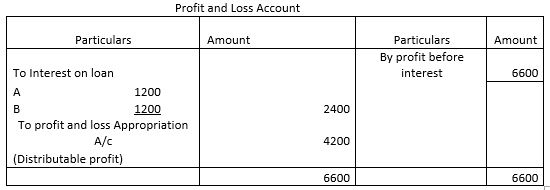Total month in a year = 12
Total months to which loan was outstanding during the year = 3

Interest on A s loan = 80000 X 6/100 X 3/12 = 1200

Interest on B s loan = 80000 X 6/100 X 3/12 = 1200

## Journal entry for commission to partner

### Accounting for partnership firms fundamentals – Question 22 : –

A and B are partners in a firm. A is to get a commission of 10 % of net profit before charging any commission. B is to get a commission of 10 % of net profit after charging all commission.Net profit before charging any commission was Rs. 88000 .Journal entry for Commission of A and B will be :

Explanation : –

A s commission as percentage of net profit before charging such commission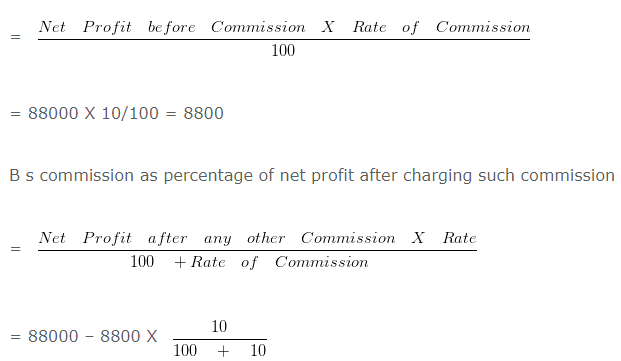= 79200 X 10/110

= 7200
A s commission A/c Dr 8800
B s commission A/c Dr 7200
To A s capital/current A/c 8800
To B s capital/current A/c 7200

## Theory Questions

### Accounting for partnership firms fundamentals – Questions 23 : –

What is the nature of partnership from legal point of view?

Explanation : –

Partnership is a separate business entity from accounting point of view, but from a legal point, partnership is not a separate
legal entity from its partners and partners are responsible for all its act.

## When appropriations are more than available profit

### Accounting for partnership firms fundamentals – Question 24 : –

A and B entered into the partnership to construct canteens in government offices in Uttarakhand.They contributed capitals of Rs. 500000 and 1000000 respectively.Their profit sharing ratio was 1 : 3 and interest allowed on capital as provided in the partnership deed was 10 % per annum.During the year ended 31st march 2016 the firm earned a profit of Rs. 300000 .The interest on capital credited into partners capital account will be:

Explanation : –

Calculation of interest on capital :

A = 500000 X 10/100 = 50000

B = 1000000 X 10/100 = 100000

Total Interest = 150000
But profit earned = 300000

A will get, 50000/150000 X 300000 = 100000

B will get, 100000/150000 X 300000 = 200000

Learn More..